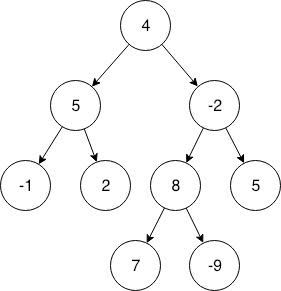# Count subtrees with Sum equal to target in binary tree

If you want to practice data structure and algorithm programs, you can go through 100+ java coding interview questions.

In this post, we will see about how to count subtrees with Sum equal to target in binary tree

## Problem

Given a Binary tree and an integer. You need to find the number of subtrees having the sum of all of its nodes equal to given Integer, that is, Target sum.

## Solution

For solving this problem, we calculate the result in postorder while returning from recursion in which we return a Pair object which contains the sum of current subtree and count of subtrees having their sum equal to given sum.

So basically, we keep on recurring to child of the current node until we finally reach to a null node where we return an empty pair class object in which sum of current subtree is zero and also the count of subtrees with sum equal to target sum equal to zero.
Result of any node or the pair to be returned will be calculated using the pair objects returned from left child and right child of the current node.
There are two things we need to calculate for current node :

• (i) SUM : Sum of current node will be the summation of left subtree sum, right subtree sum and value of
current node.
• (ii) Count : Count of subtrees with sum Equal to target sum will left count + right count and add one if the
overall sum of the current node is equal to target sum.

The time complexity of this algorithm would be similar to all other traversals like postorder, that is, O(n) , where ‘n’ is number of nodes in the tree.That’s all about Count subtrees with Sum equal to target in binary tree.

import_contacts

### You may also like:## Related Posts

•17 November

### Lowest Common Ancestor (LCA) for n-ary Tree

Table of ContentsProblemSolution If you want to practice data structure and algorithm programs, you can go through Java coding interview questions. In this post, we will see about Lowest Common Ancestor for n-ary Tree. Problem Given a n-ary tree also known as a Generic Tree and also two nodes. You need to find the Lowest common […]

•16 September

### Check if a binary tree is binary search tree or not in java

Table of ContentsFirst method:Second Method:Complete java program to check if Binary tree is binary search tree or not. If you want to practice data structure and algorithm programs, you can go through 100+ java coding interview questions. In this post, we will see how to check if given binary tree is binary search tree or not. […]

•16 April

### Delete a node from binary search tree in java

If you want to practice data structure and algorithm programs, you can go through 100+ java coding interview questions. In this post, we will see how to delete a node from binary search tree. There are two parts to it. Search the node After searching that node, delete the node. There are three cases which we […]

•14 April

### Lowest Common Ancestor (LCA) of binary tree in java

Table of ContentsRecursive Algorithm (For nodes A and B):Java Binary tree tutorial: If you want to practice data structure and algorithm programs, you can go through 100+ java coding interview questions. In this post, we will see how to find lowest common ancestor(LCA) of two nodes in binary tree. Lets understand with example. As you can […]

•13 April

### Boundary traversal of binary tree in java

Table of ContentsPrint left edge nodes (Excluding leaf nodes)Print leaf nodes:Print right edge nodes (From bottom to top) :Java Binary tree tutorial: If you want to practice data structure and algorithm programs, you can go through 100+ java coding interview questions. In this post, we will see boundary traversal of binary tree in java. Lets understand […]

•07 April

### Reverse level order traversal of binary tree in java

If you want to practice data structure and algorithm programs, you can go through 100+ java coding interview questions. This is part of java binary tree tutorial. In this post, we will see about Reverse Level Order binary tree traversal in java. In previous post, we have already seen Level order traversal. In reverse level order […]

## Subscribe to our newletter

Get quality tutorials to your inbox. Subscribe now.